Next: 2.3 The Magnetic Field Up: 2 The Characteristic Length Previous: 2.1.3 Penetration Depth for dx-y-Wave

# 2.2 The Superconducting Coherence Length

To explain numerous experimental results which deviated from the predictions of the London theory, Pippard  proposed the following general nonlocal relation for the supercurrent response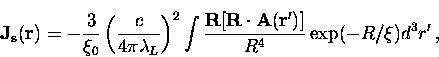(29)
where,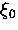is the coherence length and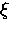is an effective coherence length related to the electron mean-free path l through the equation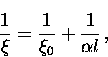(30)
whereis a constant on the order of unity. For a pure superconductor,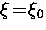.The response of the superconductor to the applied magnetic field is nonlocal, in the sense that the value of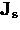measured at a point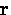depends on the value of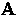throughout a volume of radiussurrounding the point.The Pippard kernel relating the kth Fourier component ofto the vector potential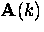can be determined from Eq. (2.30), and is given by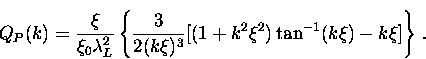(31)
QP (k) is always smaller than the London kernel QL (k). As a result, substituting the expression for QP (k) into Eq. (2.5) will always yield a value for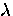which is larger than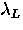.In particular, for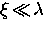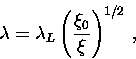(32)
and for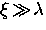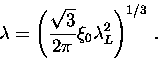(33)
Note that in the first limiting case,agrees with the London prediction in a pure superconductor. On the other hand, the second limiting case is completely independent of the electron mean-free path. A superconductor described by the first equation, is called a type-II superconductor'', whereas one that is described by the second equation is a type-I superconductor''.

Using an argument based on the uncertainty principle, Pippard estimated that the coherence length in a pure metallic superconductor is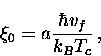(34)
where vf is the Fermi velocity and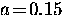.In BCS theory, the response kernel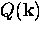is similar to that in Pippard theory. The BCS coherence length, is the range of the Fourier transform ofand is defined as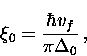(35)
where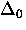is the uniform energy gap at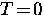. Since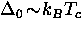, the Pippard estimate is close to the BCS coherence length. In a conventional superconductor, vf and henceis large. The bound electron pairs which make up the superfluid have a spatial extent on the order of.

The high-Tc materials differ markedly from conventional superconductors in that they have much smaller coherence lengths. Consequently, these materials are in the extreme type-II limit. The small value of the coherence length also means that the high-Tc compounds are generally in the clean limit, where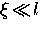. Furthermore, both fluctuation and boundary effects are much stronger in these shortsuperconductors. In a dx2-y2-wave superconductor where the energy gap is anisotropic, one must define an angle dependent coherence length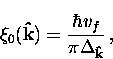(36)
where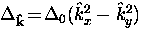andis the maximum value of the energy gap. The divergence of Eq. (2.37) along the node directions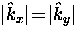means that the extreme nonlocal limit is obtained near the nodes (i.e.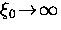). Recently, Kosztin and Legget  determined that nonlocal electrodynamics leads to a crossover from a T to a T2 dependence for the penetration depth in the Meissner state at extremely low temperatures. To observe this experimentally, one must distinguish this effect from the T2 dependence expected from sample impurities. Both the temperature and magnetic field dependence ofwill be discussed later in the thesis.Next: 2.3 The Magnetic Field Up: 2 The Characteristic Length Previous: 2.1.3 Penetration Depth for dx-y-Wave